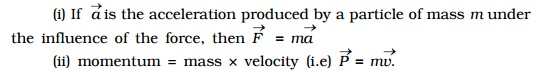Home | | Physics | Subtraction of vectors

# Subtraction of vectorsThe subtraction of a vector from another is equivalent to the addition of one vector to the negative of the other. For example vector Q ? vector P = vector Q + ( - vector P )

Subtraction of vectors

The subtraction of a vector from another is equivalent to the addition of one vector to the negative of the other.For example vector Q ? vector P = vector Q + ( - vector P )

Thus to subtract  vector P from vector Q, one has to add ? vector P with vector Q (Fig a). Therefore, to subtract  vector P from vector Q, reversed vector P is added to the  Vector Q . For this, first draw Vector  (AB ) = Vector Q and then starting from the arrow head of  Vector  Q, draw Vector (BC) = Vector  = �'(P ) and finally join the head of  �'Vector P . Vector R is the sum of  Vector Q and ? Vector P. (i.e) difference  Vector Q ? Vector P.The resultant of two vectors which are antiparallel to each other is obtained by subtracting the smaller vector from the bigger vector as shown in Fig b. The direction of the resultant vector is in the direction of the bigger vector.

Product of a vector and a scalar

Multiplication of a scalar and a vector gives a vector quantity which acts along the direction of the vector.

Examples

(i) If Vectors a is the acceleration produced by a particle of mass m under the influence of the force, then Vectors F = m . Vectors a

(ii) momentum = mass ? velocity (i.e) Vectors P = m. Vectors v.Study Material, Lecturing Notes, Assignment, Reference, Wiki description explanation, brief detail
11th 12th std standard Class Physics sciense Higher secondary school College Notes : Subtraction of vectors |